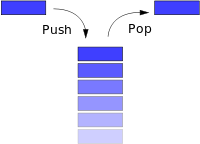# All About Class Stack in Java

0
156
• 29
• 34
• 28
• 35
•
•
•
• 12
•
•
138
Shares

## Stack in Java

The Stack class extends the Vector class. In addition to inherited methods from vector class, the Stack class contains some more extra methods. The Stack class represents a container that implements Last In First Out (LIFO) or First In Last Out(FILO) structure of Objects in Java.

That is whenever we push some object to the stack, the last is the first item we can pop out. Like other containers, we can push and pop objects. So we must cast to the appropriate type when we pop an object from Stack.

push() puts an object on the top of the stack.pop() removes and returns the top object from the stack. peek() returns the top object without removing it.

#### The structure of the Stack class is given below:

``````public class java.util.stack extends Java.util.vector{
//constructor
public stack();
//creates a stack with no elements in it.
//Methods
public boolean empty();//returns true if the stack is empty.
public synchronized Object peek();//returns the element at the top of the stack.
public synchronized Object pop();//removes the top element of the Stack and returns the element that is removed.
public Object push(Object item);//adds the item to the top of the stack.
public synchronized int search(Object itemToSearch);
}``````

### An Example of stack implementation:

``````
import java.util.*;
public class StackTest{
public static void main(String []args){
Stack st=new Stack();
st.push("hello");
st.push("hi");
st.push("Good Morning");
System.out.println("The elements of the Stack "+st);
System.out.println("The element at the top of the Stack "+st.peek());
System.out.println("The element popped out of the Stack "+st.pop());
System.out.println("The elements of the Stack after pop "+st);
System.out.println("The result of search from the Stack "+st.search("hi"));
}
}
``````

The output of the code:
\$javac StackTest.java
Note: StackTest.java uses unchecked or unsafe operations.
Note: Recompile with -Xlint: unchecked for details.
\$java -Xmx128M -Xms16M StackTest
The elements of the Stack [hello, hi, Good Morning]
The element at the top of the Stack Good Morning
The element popped out of the Stack Good Morning
The elements of the Stack after pop [hello, hi]
The result of a search from Stack 1

### Another example:

``````
import java.util.*;
public class StackTest{
public static void main(String []args){
String pathOfFile="C:Testabc.txt"
//To read a line at a time from a Stream , we need to create a BufferedReader
//object and to open a file we need to create FileReader object.
Stack st=new Stack();
String s;
//Once the BufferedReader object is created we can read file line by line by calling
//readLine() method.It breaks when end of file is reached.
pushing to stack
st.push("n"+s);
//each line in the file is read and pushed to the stack.
System.out.println("st="+st);
//treating stack as vector,it can be done without a cast.As stack is a Vector class
Vector v=st;
//we can perform all the vector related operations here
System.out.println("3rd element="+v.elementAt(2));
//poping from stack
while((!st.empty())
System.out.println("Poping="+st.pop());
}
``````

###How to Implement Stack in Java

Before we proceed further we need to understand what is Stack all about.
As per Wiki-One way of describing the stack is as a last-in, first-out (LIFO) abstract data type and linear data structure.

So our objective will be to
1.create a data structure to hold values
2.should be able to insert values in the current position
3.Should be able to remove values from last

For point#1 I will use array. This is easy to understand with Array. Later I will use some advanced concepts. So we need to create an array and a pointer that will provide me the current position of the array. let us initialize the pointer to -1. We need some control to restrict users for the pop without a push. and based on the size we can restrict the user to push beyond array capacity.
The starting of the code as follows:

``````
int array_[];
int Stack_pointer_;
int Size_=0;
public void array_set(int Size)
{
array_=new int[Size];
Stack_pointer_=-1;
}
``````

Well, now we need to implement the point-2 that It should be able to push values to the array…The Steps will be
1. Increment the pointer by one during the push.
2. Insert the value to the corresponding location.

``````
public void push(int number) {
System.out.println("Stack pointer value"+Stack_pointer_);
array_[++Stack_pointer_]=number;
}
``````

We will implement the point-3 that It should be able to pop values from the array. Here we need to decrease the pointer during the pop. So only we need to decrease the stack pointer value by one.

``````
public long pop(){
//Stack_pointer_=Stack_pointer_-1;
System.out.println("Current Stack pointer value"+Stack_pointer_);
return array_[Stack_pointer_--];
}
``````

Implementing the different Exception handling the code will look like-for Push

``````
public void push(int number) {
if (Stack_pointer_``````

Exception handling the code will look like-for POP

``````
public long  pop(int j){
//Stack_pointer_=Stack_pointer_-1;
if(Stack_pointer_==-1)
{
System.out.println("You can not pop here");
return array_[Stack_pointer_];
}
else
{
System.out.println("Poping "+j+array_[j]);
return  array_[Stack_pointer_--];
}

}
``````

The final code will look like—

``````
import java.io.PrintStream;
import java.text.SimpleDateFormat;
import java.util.Date;
import javax.swing.JOptionPane;
public class Calculator {
int array_[];
int Stack_pointer_;
int Size_=0;
public void push(int number) {
if (Stack_pointer_=0;j--)
{
System.out.println("Poping"+j);
calc.pop(j);
}
}
}
``````
Don't miss out!

Receive top technical news, lesson ideas, travel tips and more!

Give it a try. You can unsubscribe at any time.